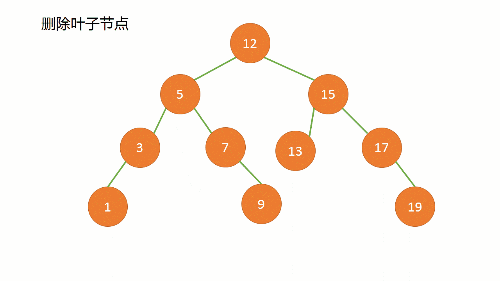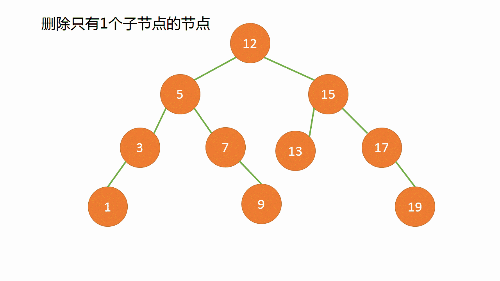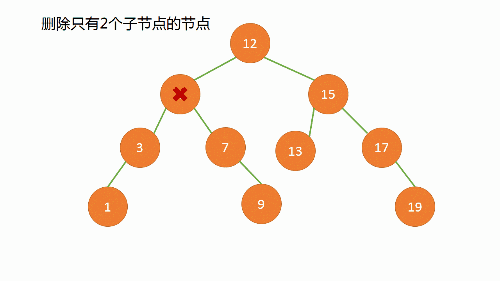二叉查找树节点的删除

简介

（1）所有左子树中的节点小于等于根节点
（2）所有右子树中的节点大于等于根节点
（3）对于任意节点满足（1）（2）

问题分析

（1）删除叶子节点（2）被删除的节点只有一个子节点（3）被删除的节点左右子树都有

• 找出左子树中最大或者右子树中最小的值val
• 将当前节点的值替换为val
• 在左子树或者右子树中找到val删除

Note:
由于二叉查找树的性质，如果将当前节点替换为左子树中最大的或者右子树中最小的一定不会破坏二叉查找树的结构。代码

public TreeNode deleteNode(TreeNode root, int key) {

if(root == null){
return root;
}
if(key < root.val){
root.left = deleteNode(root.left, key);
return root;
}
if(key > root.val){
root.right = deleteNode(root.right, key);
return root;
}
//开始执行删除操作
//(1)删除根节点
if(root.left == null && root.right == null){
root = null;
return root;
}
//(2)只有一个child,只有左子树
if(root.left == null && root.right != null){
root = root.right;
return root;
}
//(2)只有一个child,只有右子树
if(root.right == null && root.left != null){
root = root.left;
return root;
}
//(3)有两个child
if(root.left != null && root.right != null){
//挑选左子树中最大的或者右子树中最小的，替换当前节点，再将替换的节点置空
int val = findMaxInLeftTree(root.left);
root.val = val;
root.left = deleteNode(root.left, val);
return root;
}
return root;

}
//找到左子树中最大的值
private int findMaxInLeftTree(TreeNode left) {
if(left == null){
return 0;
}
if(left.right == null){
return left.val;
}
if(left.right == null && left.left == null){
return left.val;
}
return findMaxInLeftTree(left.right);
}
}